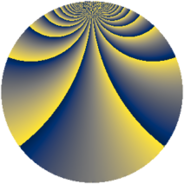# Properties

 Label 230.2.lLevel $230$ Weight $2$ Character orbit 230.l Rep. character $\chi_{230}(7,\cdot)$ Character field $\Q(\zeta_{44})$ Dimension $240$ Newform subspaces $1$ Sturm bound $72$ Trace bound $0$

# Related objects

## Defining parameters

 Level: $$N$$ $$=$$ $$230 = 2 \cdot 5 \cdot 23$$ Weight: $$k$$ $$=$$ $$2$$ Character orbit: $$[\chi]$$ $$=$$ 230.l (of order $$44$$ and degree $$20$$) Character conductor: $$\operatorname{cond}(\chi)$$ $$=$$ $$115$$ Character field: $$\Q(\zeta_{44})$$ Newform subspaces: $$1$$ Sturm bound: $$72$$ Trace bound: $$0$$

## Dimensions

The following table gives the dimensions of various subspaces of $$M_{2}(230, [\chi])$$.

Total New Old
Modular forms 800 240 560
Cusp forms 640 240 400
Eisenstein series 160 0 160

## Trace form

 $$240q - 8q^{3} - 8q^{6} + O(q^{10})$$ $$240q - 8q^{3} - 8q^{6} - 8q^{12} + 16q^{13} + 24q^{16} - 72q^{18} - 80q^{23} - 8q^{26} + 16q^{27} - 44q^{28} + 24q^{31} - 44q^{33} - 8q^{35} - 32q^{36} - 88q^{37} - 24q^{41} - 8q^{46} - 80q^{47} + 8q^{48} + 8q^{50} + 16q^{52} - 44q^{56} - 88q^{57} - 176q^{61} - 32q^{62} - 176q^{66} + 24q^{70} - 336q^{71} + 16q^{72} - 88q^{73} - 36q^{75} - 24q^{77} + 40q^{78} - 136q^{81} + 40q^{82} - 100q^{85} - 44q^{86} + 40q^{87} + 8q^{92} + 56q^{93} + 52q^{95} + 8q^{96} + 132q^{97} + 24q^{98} + O(q^{100})$$

## Decomposition of $$S_{2}^{\mathrm{new}}(230, [\chi])$$ into newform subspaces

Label Dim. $$A$$ Field CM Traces $q$-expansion
$$a_2$$ $$a_3$$ $$a_5$$ $$a_7$$
230.2.l.a $$240$$ $$1.837$$ None $$0$$ $$-8$$ $$0$$ $$0$$

## Decomposition of $$S_{2}^{\mathrm{old}}(230, [\chi])$$ into lower level spaces

$$S_{2}^{\mathrm{old}}(230, [\chi]) \cong$$ $$S_{2}^{\mathrm{new}}(115, [\chi])$$$$^{\oplus 2}$$